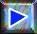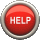﻿ The percentage of elements of a substance# The percentage of elements

## Tutorial 1Calculate the percentage of hydrogen in water. First, the molar mass $M$ of the water:

For answers, use (possibly several times) the arrows ↑ Down! and ↓ Up! Complete please this question before moving on to the next one!

$M$ $=$ $18\frac{g}{mol}$

Then the sum $S$ of the atomic masses of hydrogen:

$S$ $=$ $2\cdot M_{H}$ $=$ $2\frac{g}{mol}$

Then the percentage of hydrogen:

$\%_{H}$ $=$ $\frac{S\cdot 100}{M}$ $=$ $11.1$